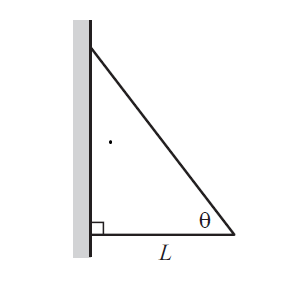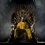# Exercise 2.36 Two sticks and a wallTwo sticks are connected, with hinges, to each other and to a wall. The bottom stick is horizontal and has length $L$, and the sticks make an angle of $\theta$ with each other, as shown in Figure above. If both sticks have the same mass per unit length, $\rho$, find the horizontal and vertical components of the force that the wall exerts on the top hinge.

Also show that the magnitude goes to infinity for both $\theta$$0$ and $\theta$$\frac{\pi}{2}$Note by Beakal Tiliksew
7 years, 1 month ago

This discussion board is a place to discuss our Daily Challenges and the math and science related to those challenges. Explanations are more than just a solution — they should explain the steps and thinking strategies that you used to obtain the solution. Comments should further the discussion of math and science.

When posting on Brilliant:

• Use the emojis to react to an explanation, whether you're congratulating a job well done , or just really confused .
• Ask specific questions about the challenge or the steps in somebody's explanation. Well-posed questions can add a lot to the discussion, but posting "I don't understand!" doesn't help anyone.
• Try to contribute something new to the discussion, whether it is an extension, generalization or other idea related to the challenge.

MarkdownAppears as
*italics* or _italics_ italics
**bold** or __bold__ bold
- bulleted- list
• bulleted
• list
1. numbered2. list
1. numbered
2. list
Note: you must add a full line of space before and after lists for them to show up correctly
paragraph 1paragraph 2

paragraph 1

paragraph 2

[example link](https://brilliant.org)example link
> This is a quote
This is a quote
    # I indented these lines
# 4 spaces, and now they show
# up as a code block.

print "hello world"
# I indented these lines
# 4 spaces, and now they show
# up as a code block.

print "hello world"
MathAppears as
Remember to wrap math in $$ ... $$ or $ ... $ to ensure proper formatting.
2 \times 3 $2 \times 3$
2^{34} $2^{34}$
a_{i-1} $a_{i-1}$
\frac{2}{3} $\frac{2}{3}$
\sqrt{2} $\sqrt{2}$
\sum_{i=1}^3 $\sum_{i=1}^3$
\sin \theta $\sin \theta$
\boxed{123} $\boxed{123}$

Sort by:alt text

In the above figure, I have marked all the forces that are present in the system. Let the masses of the two rods be $\displaystyle m_1$ and $\displaystyle m_2$.

Thus,
$m_1 = \rho L\sec\theta$ $m_2 = \rho L$

Considering the torque about the point A,
$h_1(L\tan\theta) = (m_1+m_2)g\frac{L}{2}$

$h_1(L\tan\theta) = \rho L (1+\sec\theta)g\frac{L}{2}$

$\boxed{h_1 = \rho\frac{1+\sec\theta}{\tan\theta}\frac{gL}{2}}$

Ill do the vertical force later..Ill post it as a comment

- 7 years, 1 month ago

Nice explanation

- 7 years, 1 month ago

Why are there no forces at the hinge joining the 2 sticks ??

- 5 years, 11 months ago

There are forces at the hinge joining the sticks, but they are internal forces. So, they cancel each other off..

- 5 years, 11 months ago

Got it ! Thanks a lot !!

- 5 years, 11 months ago

do you know any good book with exersises in rotational dynamics ;

- 6 years, 6 months ago

Try IE Irodov or Krotov

- 6 years, 6 months ago

The vertical force can be found using the pivot point B. It is -(rholg/2)(1+2sec(theta)).

- 3 years ago